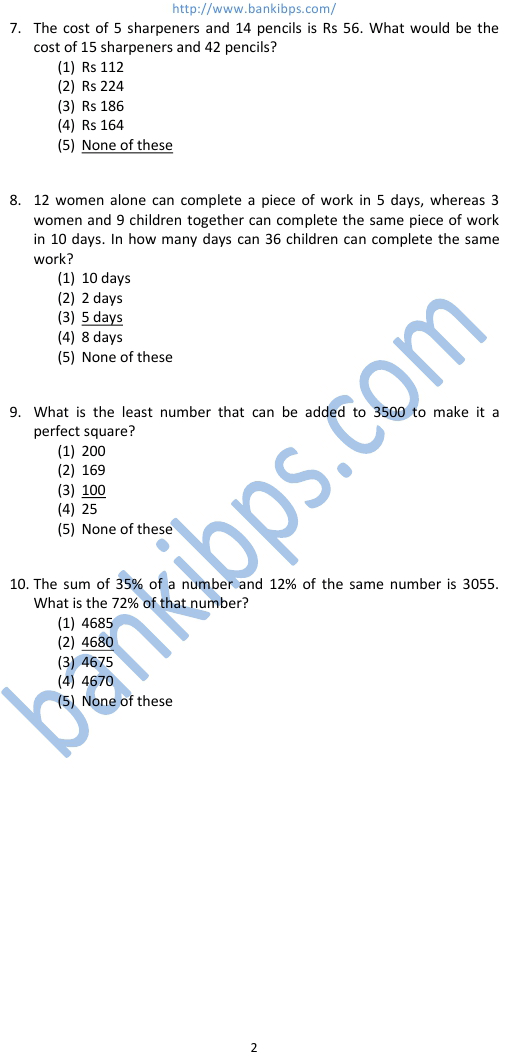# Exam Pattern for IBPS Clerk

Direction (1 – 5): Find out the approximate value which should replace the question mark (?) in the following questions. (You are not expected to find out the exact value) 953.7 ÷ 950.9989 = 95? 1.9 3 2.99 3.6 2.7 exam pattern for ibps clerk v1000 + 3.001/4.987 of 1891.992 = ? 2500 1230 1640 1525 2130 0.0004 ÷ 0.0001 x 36.000009 = ? 0.10 1.45 145 14.5 1450 137 % of 12345 = ? 17000 15000 1500 14300 900 3739 + 164 x 27 = ? 105400 4000 8200 690 6300 The average of four consecutive even numbers P, Q, R and S respectively is 55. What is the product of Q and S? 3123 3135 3132 3225 None of these
Aptitude